# 数据挖掘-支持向量机(SVM)+代码实现# 从例子出发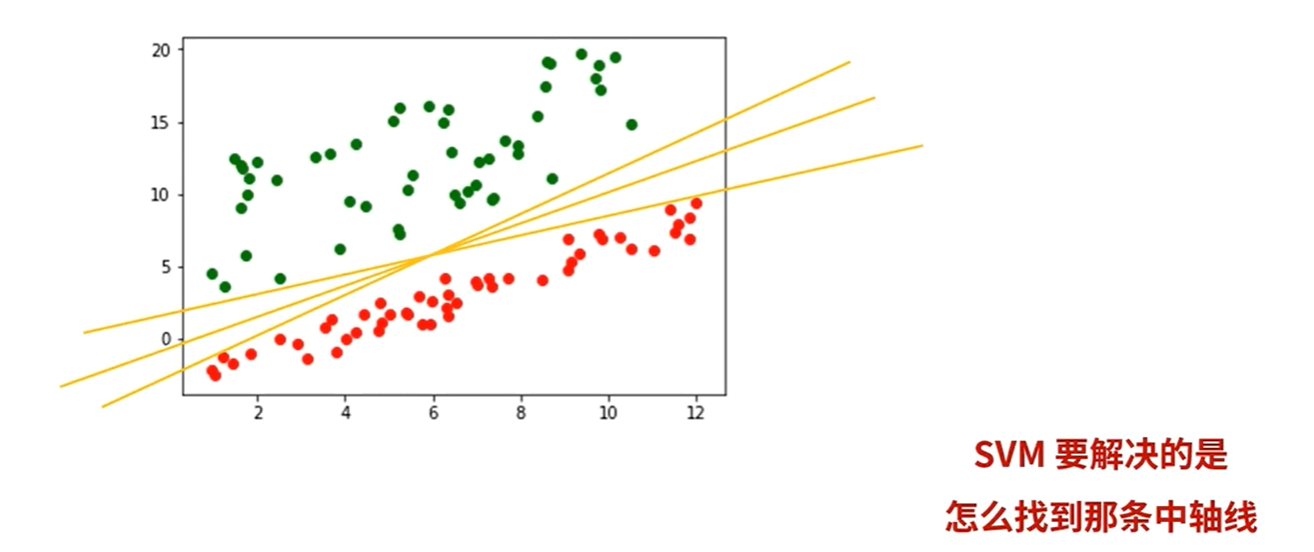# 算法原理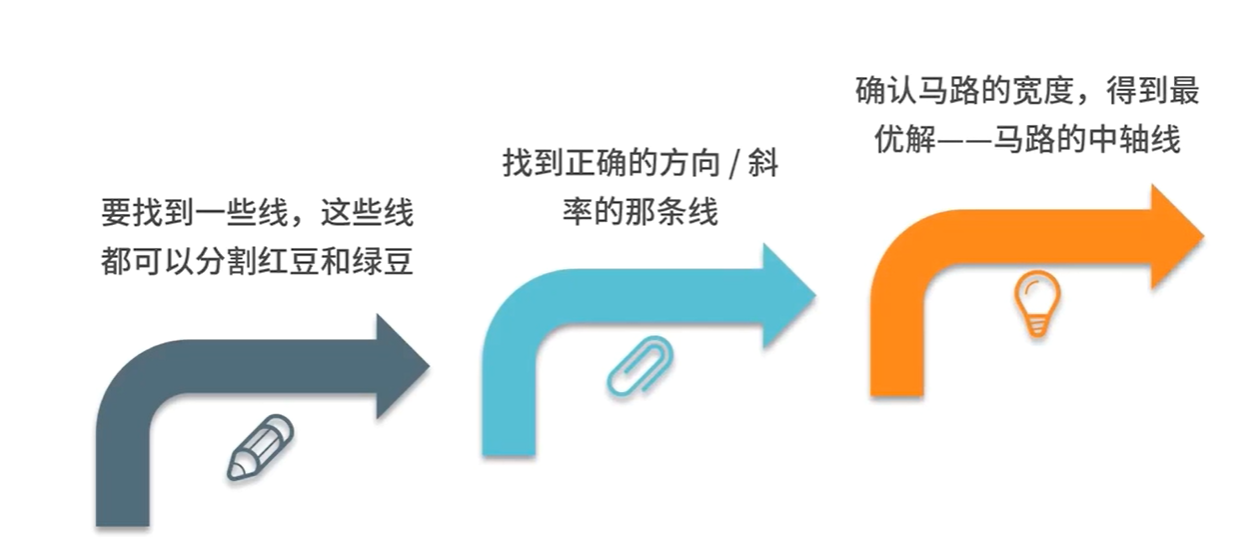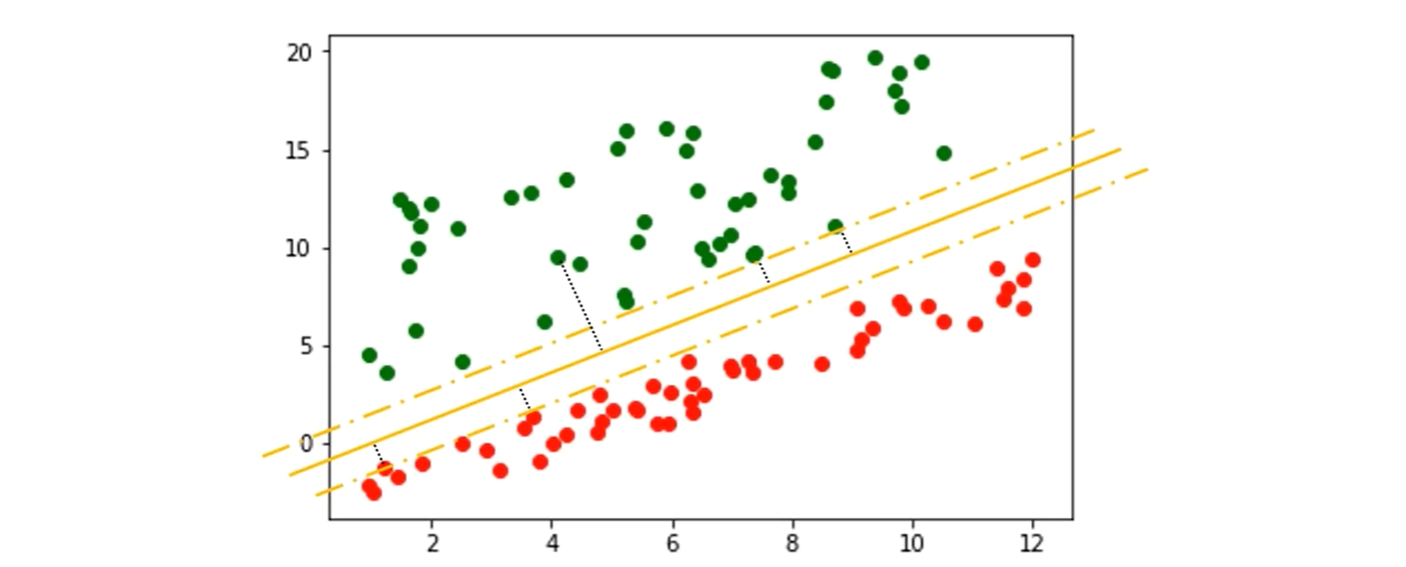# 超平面# 如何处理不清晰的边界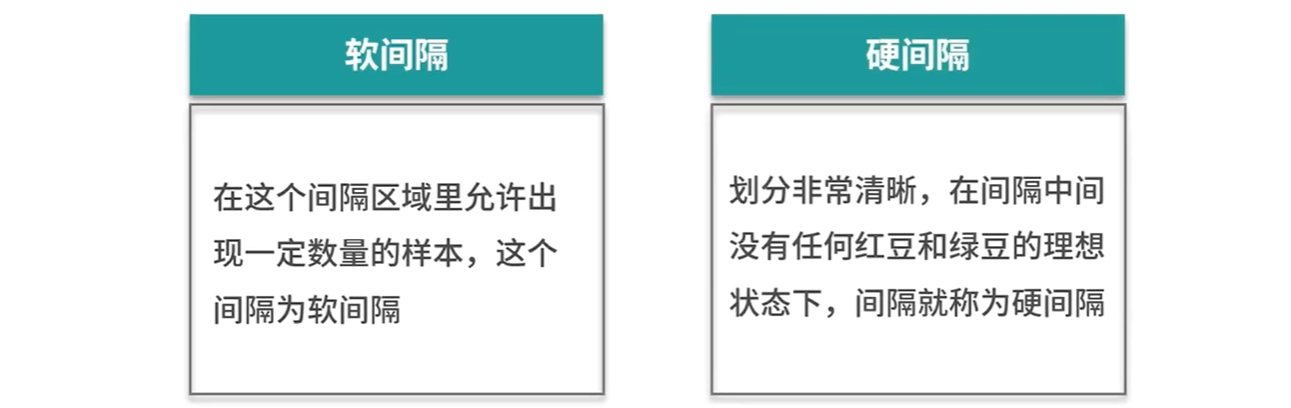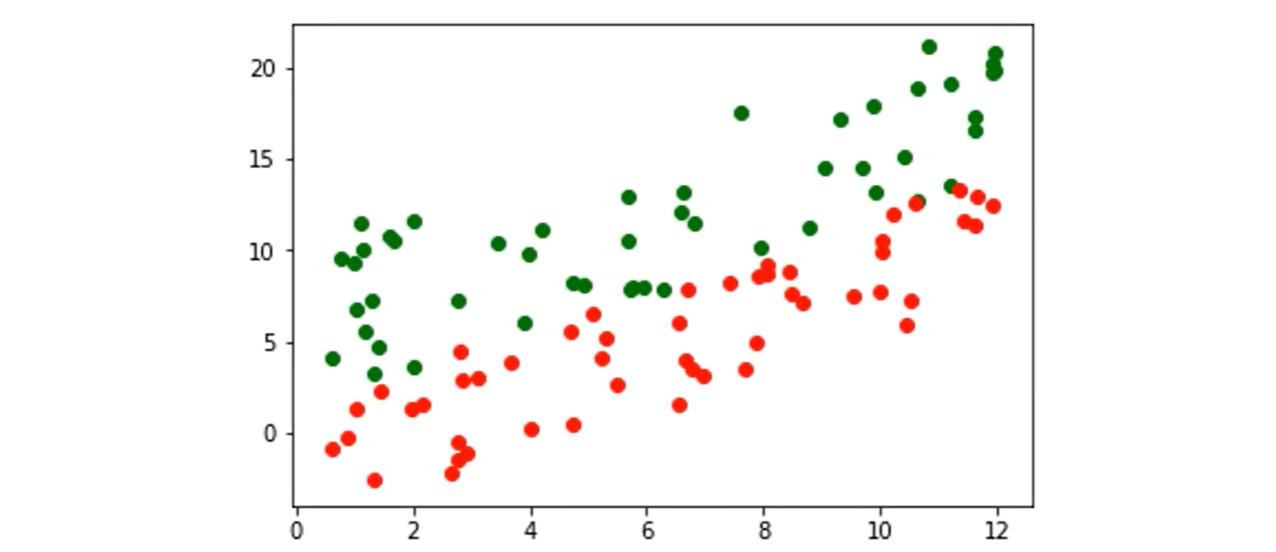# 非线性可分的情况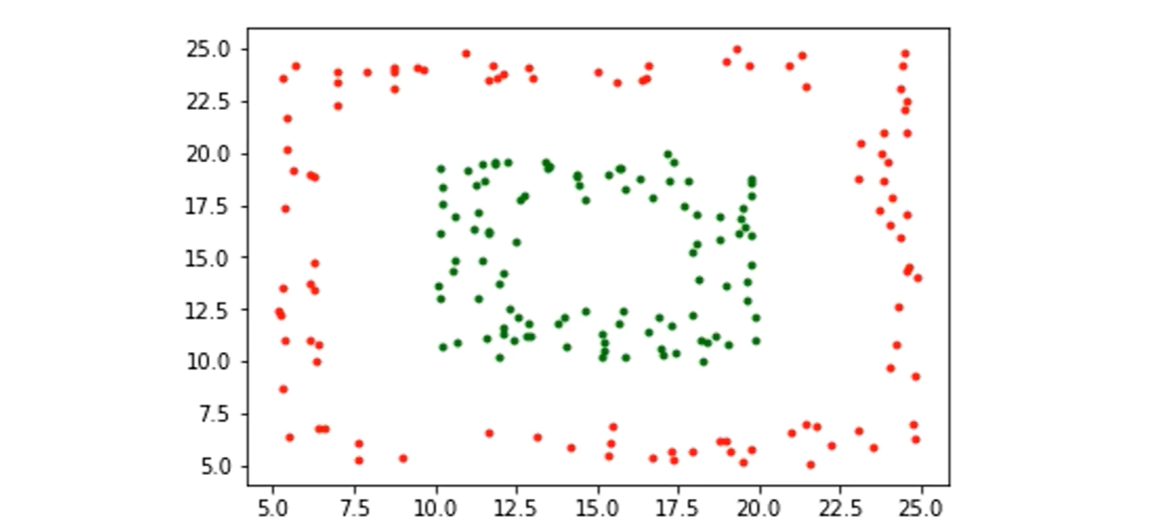SVM中采取的办法是：

# 常见的核函数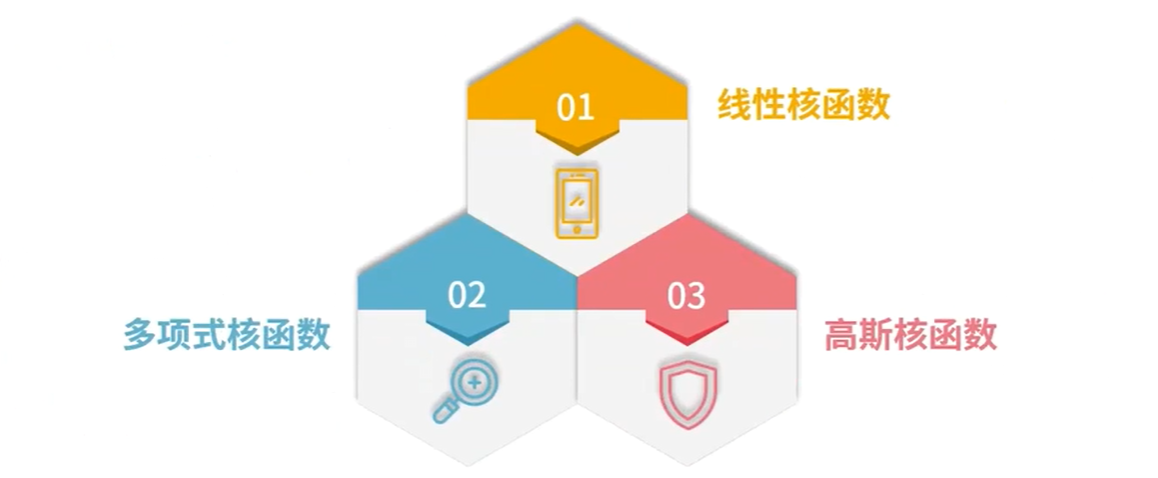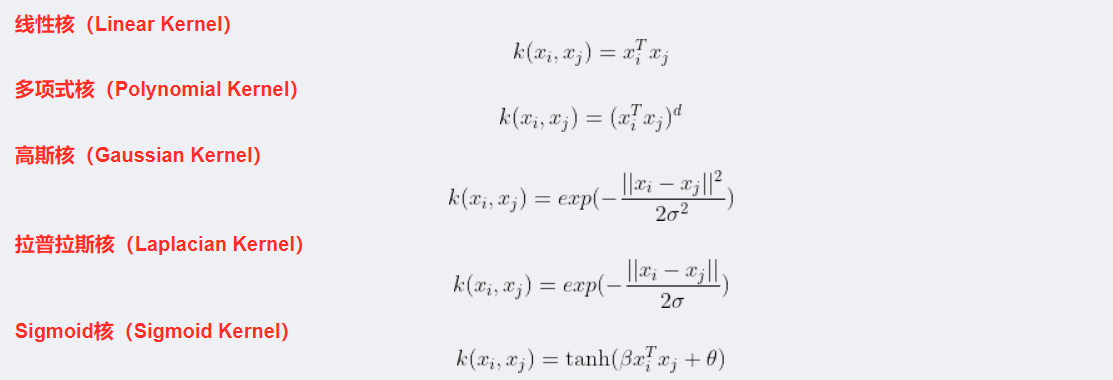#支持向量机算法可以解决小样本情况下的机器学习问题，简化了通常的分类和回归等问题。

#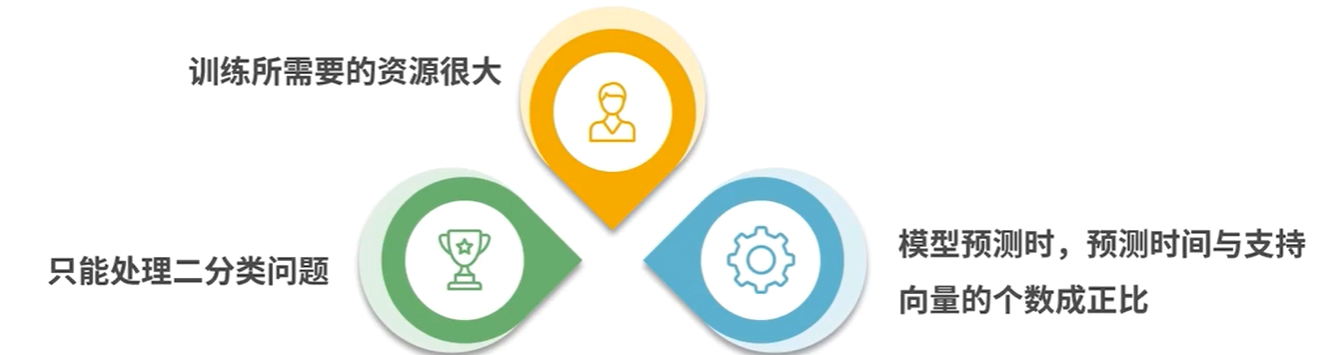SVM算法效果与核函数的选择关系很大，往往需要尝试多种核函数，即使选择了效果比较好的高斯核函数，也要调参选择恰当的参数。另一方面就是现在常用的SVM理论都是使用固定惩罚系数C，但正负样本的两种错误造成的损失是不一样的。

# 代码的实现

``````from sklearn import datasets
from sklearn import svm
#引入svm包
import numpy as np
np.random.seed(0)
iris_x=iris.data
iris_y=iris.target
indices= np.random.permutation(len(iris_x))
iris_x_train = iris_x[indices[:-10]]
iris_y_train= iris_y[indices[:-10]]
iris_x_test = iris_x[indices[-10:]]
iris_y_test = iris_y[indices[-10:]]
#使用线性核SVC是分类支持向量机的意思，另外还有SVR是回归支持向量机
clf = svm.SVC(kernel = 'linear')
clf.fit(iris_x_train,iris_y_train)#拟合
#调用该对象的测试方法，主要接收一个参数:测试数据集``````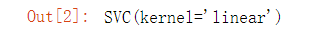``````iris_x__test = iris_x[indices[-10:]]
iris_y_test = iris_y[indices[-10:]]
#使用线性核SVC是分类支持向量机的意思,另外还有SVR是回归支持向量机
clf = svm.SVC(kernel= 'linear')
clf.fit(iris_x_train,iris_y_train) #拟合
#调用该对象的测试方法,主要接收一个参数:测试数据集
iris_y_predict= clf.predict(iris_x_test)
#调用该对象的打分方法,计算出准确率
score=clf.score(iris_x_test,iris_y_test,sample_weight=None)
print('iris_y_predict=')
print(iris_y_predict)
print('iris_y_test= ')
print(iris_y_test)
print('Accuracy:"',score)``````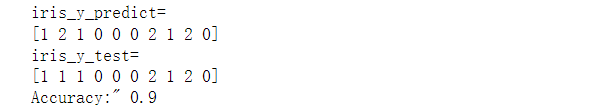(0)